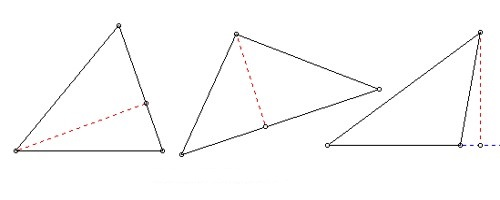Altitude of a Triangle

In geometry, an altitude of a triangle is a line segment through a vertex and perpendicular to a line containing the base. The definition of the altitude of a triangle is a line that extends from one vertex of a triangle perpendicular to the opposite side.

Sometimes the opposite side isn’t quite long enough to draw an altitude, so we are allowed to extend it to make an altitude possible. This line containing the opposite side is called the extended base of the altitude. The intersection of the extended base and the altitude is called the foot of the altitude. A triangle, therefore, has three possible altitudes. The altitude is the shortest distance from a vertex to its opposite side. The length of the altitude, often simply called “the altitude”, is the distance between the extended base and the vertex. The process of drawing the altitude from the vertex to the foot is known as dropping the altitude at that vertex. It is a special case of orthogonal projection.Fig: Some examples of the altitude of a triangle

Definition: Any side of a triangle can be assumed as its base. Since a triangle has three sides, hence it can have three bases. The altitude of a triangle is defined as a perpendicular drawn from any vertex (a point where two sides of a triangle meet) on to the opposite side (base)of that triangle, i.e. an altitude may be referred as a line segment which passes through any vertex and forms the right angle with the edge opposite to this vertex.

An altitude is a line which passes through a vertex of a triangle and meets the opposite side at right angles. A triangle has three altitudes. An interesting fact is that the three altitudes always pass through a common point called the orthocenter of the triangle. The length of a perpendicular from a side of the triangle to the opposite vertex. This is often used to calculate the area of a triangle.

Properties

(1) In an acute triangle, all the three altitudes fall inside the triangle.

(2) In the obtuse triangle, two altitudes fall outside, while one altitude falls inside the triangle. Basically, the altitude corresponding to the vertex with obtuse angle is inside and other altitudes corresponding to the acute angles are outside.

(3) The two altitudes of a right triangle are actually the perpendicular legs of the triangle itself, while the third altitude which is perpendicular to hypotenuse falls inside the triangle dividing the triangle into two congruent triangles which are congruent to the original triangle.

(4) The equilateral triangle has all three altitudes of equal length.

(5) In an isosceles triangle, two altitudes have equal length.

(6) The lengths of all the altitudes of a scalene triangle are different.

Information Source: